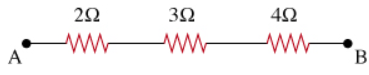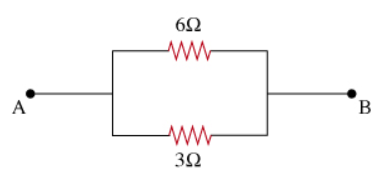# Problem: (a) For the combination of resistors shown, find the equivalent resistance between points A and B. Express your answer in Ohms.(b) If the circuit is as shown below, find the equivalent resistance between points A and B.

###### FREE Expert Solution

(a) For resistors in series:

$\begin{array}{rcl}{\mathbf{R}}_{\mathbf{e}\mathbf{q}}& \mathbf{=}& {\mathbf{R}}_{\mathbf{1}}\mathbf{+}{\mathbf{R}}_{\mathbf{2}}\mathbf{+}{\mathbf{R}}_{\mathbf{3}}\\ & \mathbf{=}& \mathbf{2}\mathbf{\Omega }\mathbf{+}\mathbf{3}\mathbf{\Omega }\mathbf{+}\mathbf{4}\mathbf{\Omega }\end{array}$

96% (5 ratings)###### Problem Details

(a) For the combination of resistors shown, find the equivalent resistance between points A and B. Express your answer in Ohms.(b) If the circuit is as shown below, find the equivalent resistance between points A and B.